# [Python图像处理] 七.图像阈值化处理及算法对比

PS：请求帮忙点个Star，哈哈，第一次使用Github，以后会分享更多代码，一起加油。

1.阈值化
2.二进制阈值化
3.反二进制阈值化
4.截断阈值化
5.反阈值化为0
6.阈值化为0

PS：文章参考自己以前系列图像处理文章及OpenCV库函数，同时部分参考网易云视频，推荐大家去学习。同时，本篇文章涉及到《计算机图形学》基础知识，请大家下来补充。

PSS：2019年1~2月作者参加了CSDN2018年博客评选，希望您能投出宝贵的一票。我是59号，Eastmount，杨秀璋。投票地址：https://bss.csdn.net/m/topic/blog_star2018/index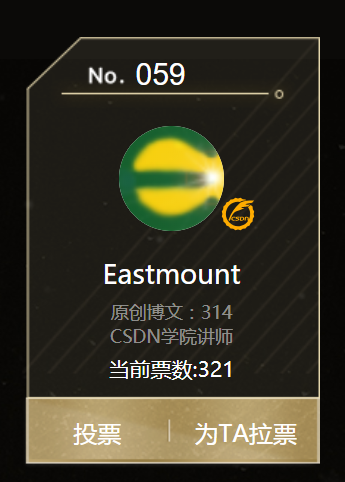# 一. 阈值化

（注：该部分参考作者的论文《基于苗族服饰的图像锐化和边缘提取技术研究》）

$\begin{cases} Y=0，gray=T\\ \end{cases}$

Python OpenCV中提供了阈值函数threshold()实现二值化处理，其公式及参数如下图所示：
retval, dst = cv2.threshold(src, thresh, maxval, type)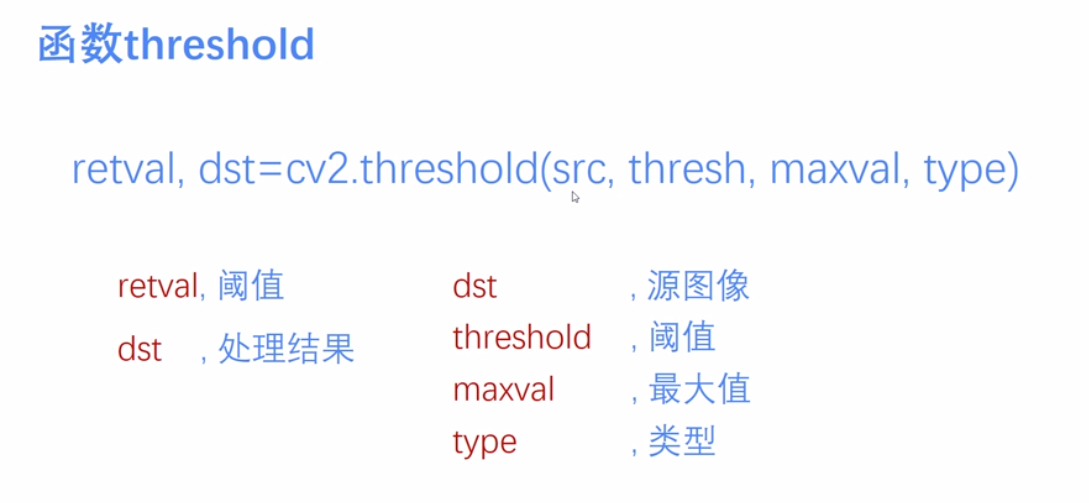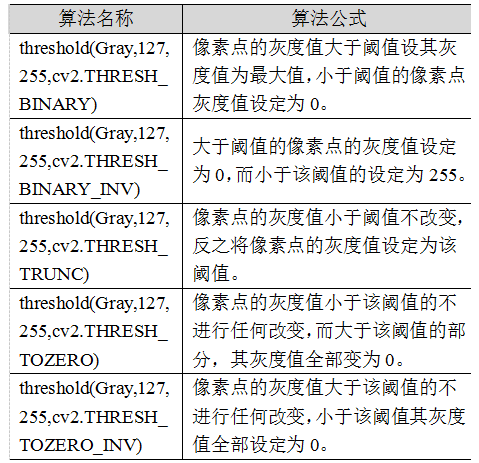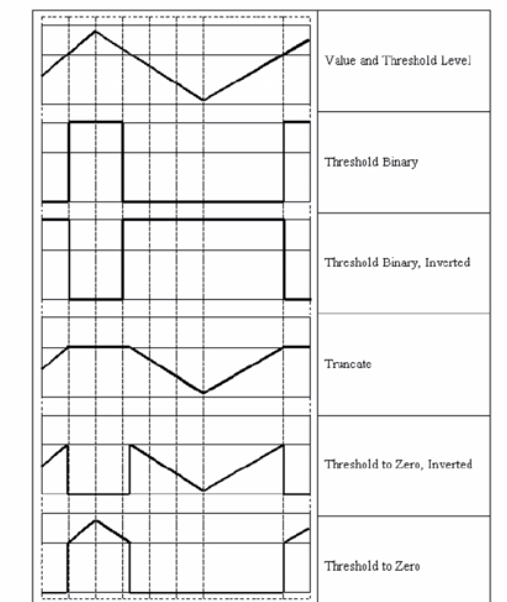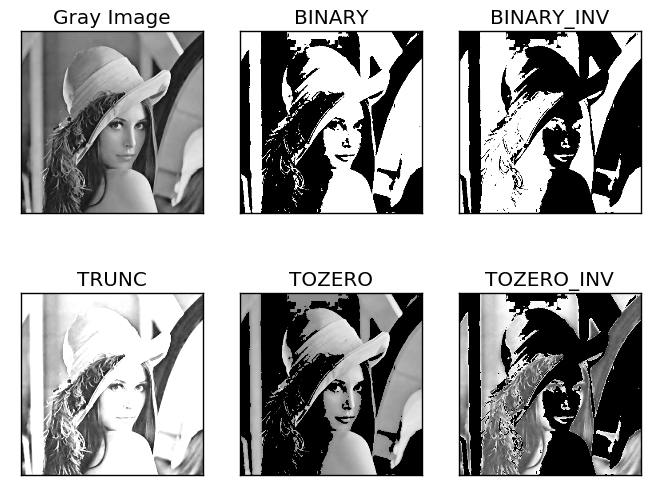# 二. 二进制阈值化

$dst(x,y) = \begin{cases} maxVal， if src(x,y)>thresh\\ 0，otherwise\\ \end{cases}$
(1) 大于等于127的像素点的灰度值设定为最大值（如8位灰度值最大为255）
(2) 灰度值小于127的像素点的灰度值设定为0

#encoding:utf-8
import cv2
import numpy as np

#读取图片

#灰度图像处理
GrayImage = cv2.cvtColor(src,cv2.COLOR_BGR2GRAY)

#二进制阈值化处理
r, b = cv2.threshold(GrayImage, 127, 255, cv2.THRESH_BINARY)
print r

#显示图像
cv2.imshow("src", src)
cv2.imshow("result", b)

#等待显示
cv2.waitKey(0)
cv2.destroyAllWindows()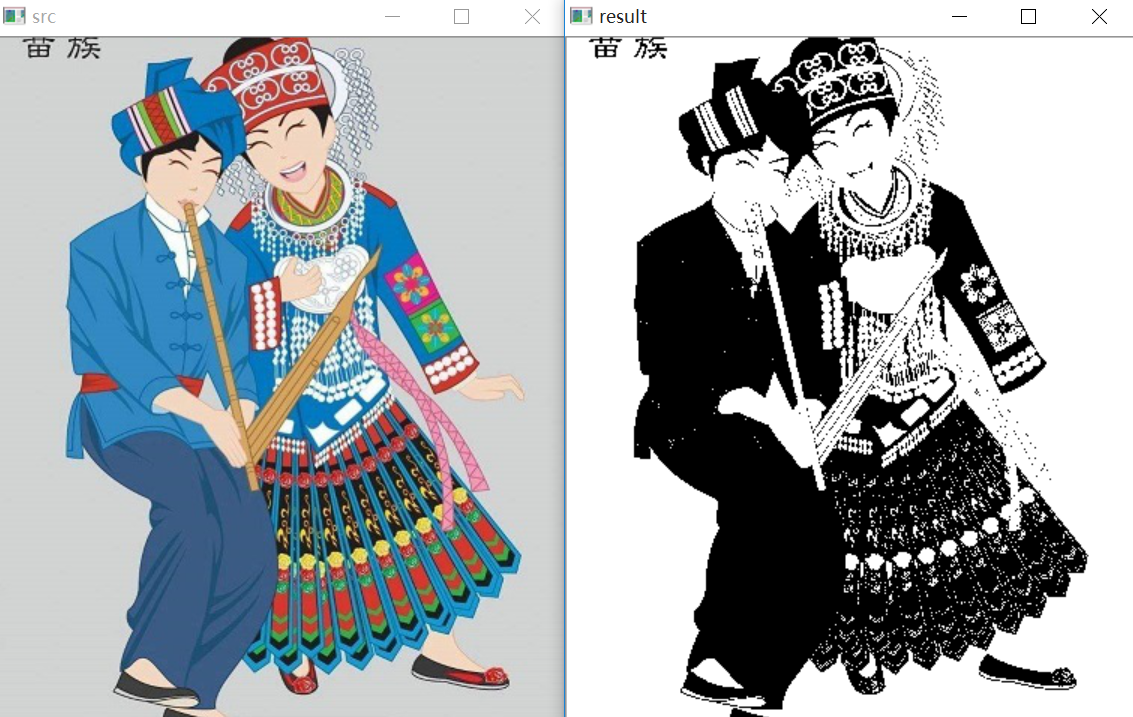# 三. 反二进制阈值化

$dst(x,y) = \begin{cases} 0， if src(x,y)>thresh\\ maxVal，otherwise\\ \end{cases}$
(1) 大于127的像素点的灰度值设定为0（以8位灰度图为例）
(2) 小于该阈值的灰度值设定为255

#encoding:utf-8
import cv2
import numpy as np

#读取图片

#灰度图像处理
GrayImage = cv2.cvtColor(src,cv2.COLOR_BGR2GRAY)

#反二进制阈值化处理
r, b = cv2.threshold(GrayImage, 127, 255, cv2.THRESH_BINARY_INV)
print r

#显示图像
cv2.imshow("src", src)
cv2.imshow("result", b)

#等待显示
cv2.waitKey(0)
cv2.destroyAllWindows()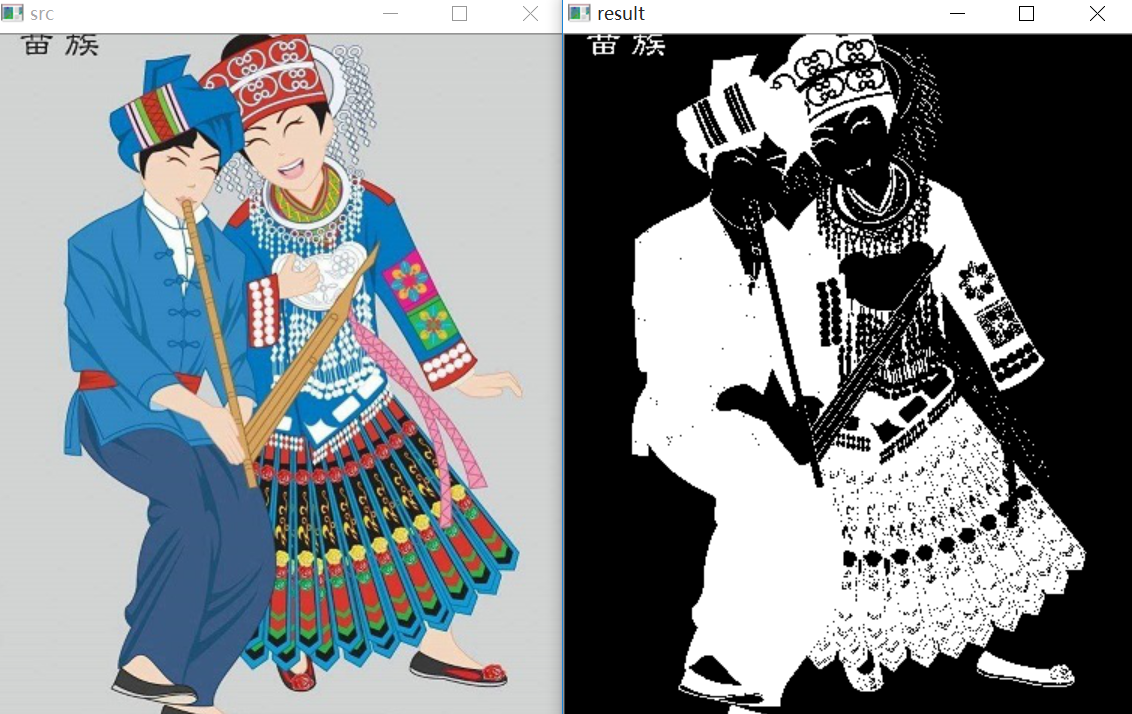# 四. 截断阈值化

$dst(x,y) = \begin{cases} threshold， if src(x,y)>thresh\\ src(x,y)，otherwise\\ \end{cases}$
(1) 大于等于127的像素点的灰度值设定为该阈值127
(2) 小于该阈值的灰度值不改变

#encoding:utf-8
import cv2
import numpy as np

#读取图片

#灰度图像处理
GrayImage = cv2.cvtColor(src,cv2.COLOR_BGR2GRAY)

#截断阈值化处理
r, b = cv2.threshold(GrayImage, 127, 255, cv2.THRESH_TRUNC)
print r

#显示图像
cv2.imshow("src", src)
cv2.imshow("result", b)

#等待显示
cv2.waitKey(0)
cv2.destroyAllWindows()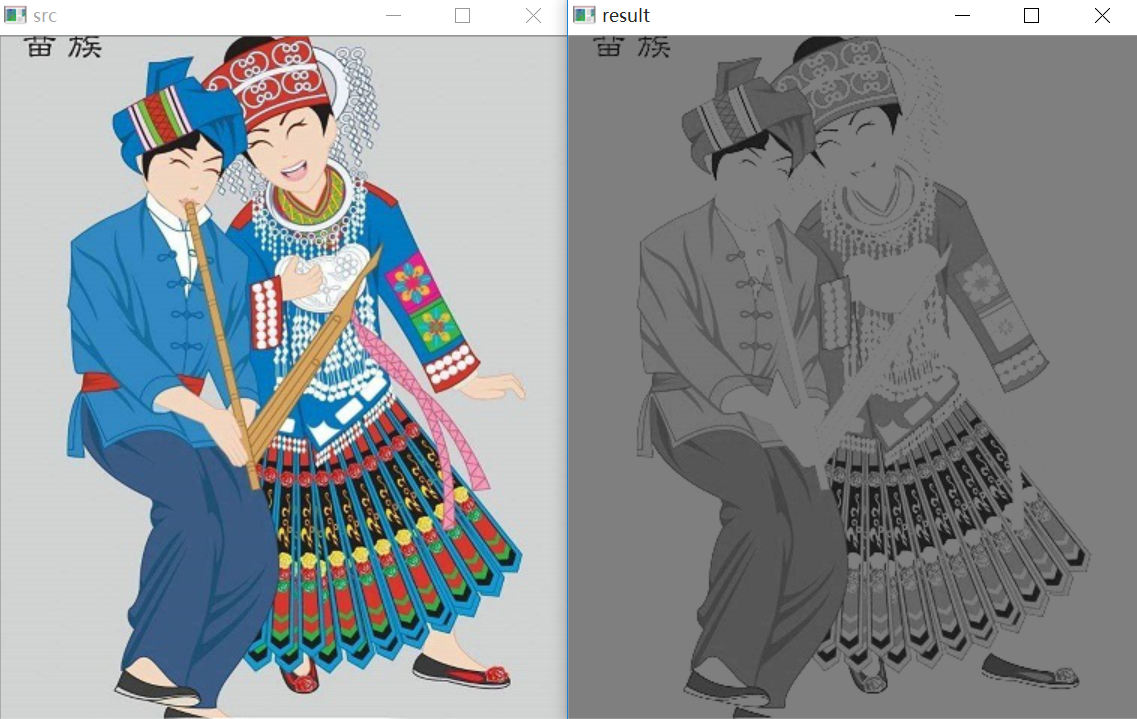# 五. 反阈值化为0

$dst(x,y) = \begin{cases} 0， if src(x,y)>thresh\\ src(x,y)，otherwise\\ \end{cases}$
(1) 大于等于阈值127的像素点变为0
(2) 小于该阈值的像素点值保持不变

#encoding:utf-8
import cv2
import numpy as np

#读取图片

#灰度图像处理
GrayImage = cv2.cvtColor(src,cv2.COLOR_BGR2GRAY)

#反阈值化为0处理
r, b = cv2.threshold(GrayImage, 127, 255, cv2.THRESH_TOZERO_INV)
print r

#显示图像
cv2.imshow("src", src)
cv2.imshow("result", b)

#等待显示
cv2.waitKey(0)
cv2.destroyAllWindows()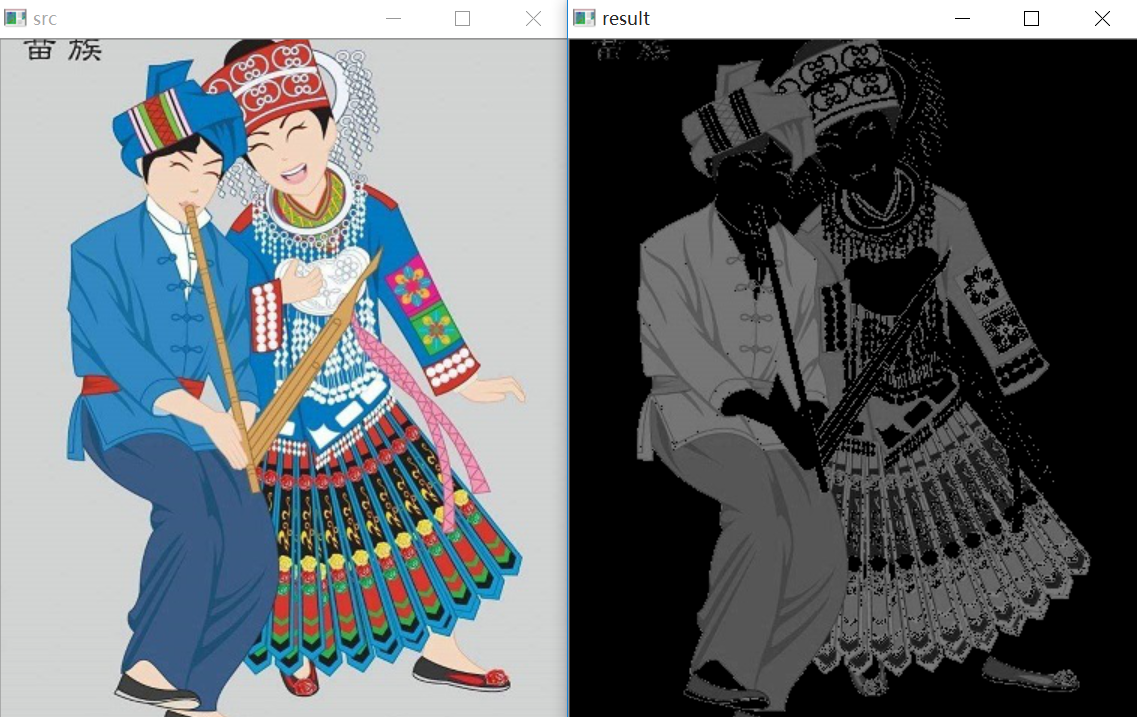# 六. 阈值化为0

$dst(x,y) = \begin{cases} src(x,y)， if src(x,y)>thresh\\ 0，otherwise\\ \end{cases}$
(1) 大于等于阈值127的像素点，值保持不变
(2) 小于该阈值的像素点值设置为0

#encoding:utf-8
import cv2
import numpy as np

#读取图片

#灰度图像处理
GrayImage = cv2.cvtColor(src,cv2.COLOR_BGR2GRAY)

#阈值化为0处理
r, b = cv2.threshold(GrayImage, 127, 255, cv2.THRESH_TOZERO)
print r

#显示图像
cv2.imshow("src", src)
cv2.imshow("result", b)

#等待显示
cv2.waitKey(0)
cv2.destroyAllWindows()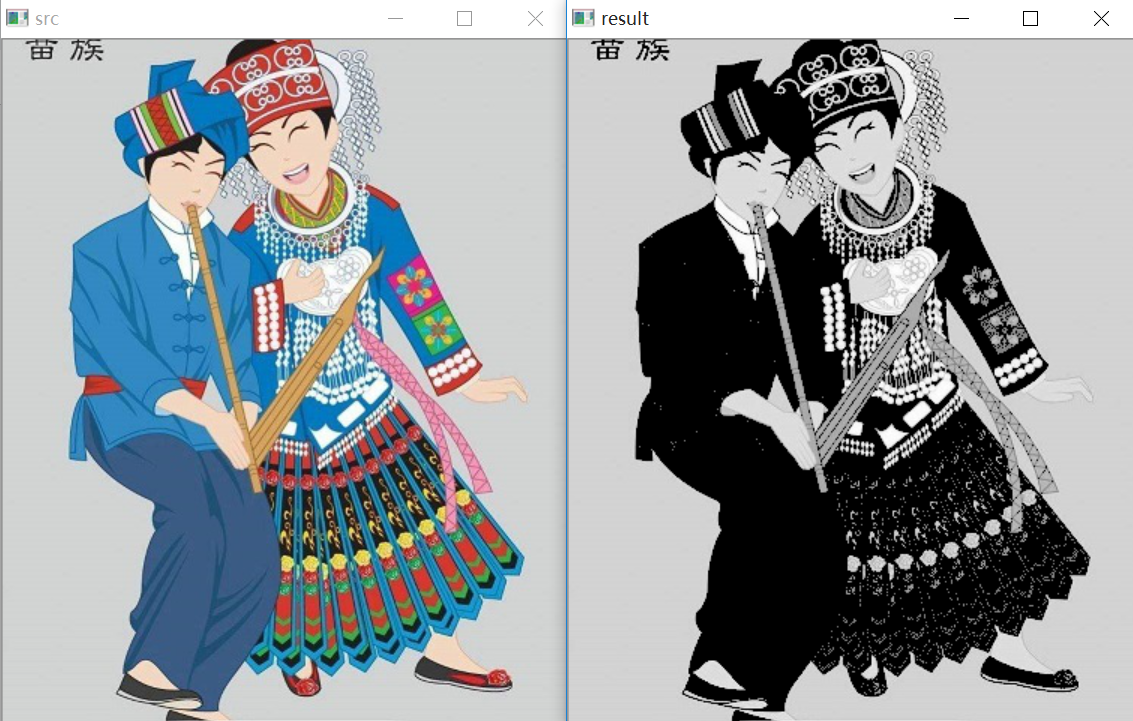#encoding:utf-8
import cv2
import numpy as np
import matplotlib.pyplot as plt

#读取图像
lenna_img = cv2.cvtColor(img,cv2.COLOR_BGR2RGB)
GrayImage=cv2.cvtColor(img,cv2.COLOR_BGR2GRAY)

#阈值化处理
ret,thresh1=cv2.threshold(GrayImage,127,255,cv2.THRESH_BINARY)
ret,thresh2=cv2.threshold(GrayImage,127,255,cv2.THRESH_BINARY_INV)
ret,thresh3=cv2.threshold(GrayImage,127,255,cv2.THRESH_TRUNC)
ret,thresh4=cv2.threshold(GrayImage,127,255,cv2.THRESH_TOZERO)
ret,thresh5=cv2.threshold(GrayImage,127,255,cv2.THRESH_TOZERO_INV)

#显示结果
titles = ['Gray Image','BINARY','BINARY_INV','TRUNC','TOZERO','TOZERO_INV']
images = [GrayImage, thresh1, thresh2, thresh3, thresh4, thresh5]
for i in xrange(6):
plt.subplot(2,3,i+1),plt.imshow(images[i],'gray')
plt.title(titles[i])
plt.xticks([]),plt.yticks([])
plt.show()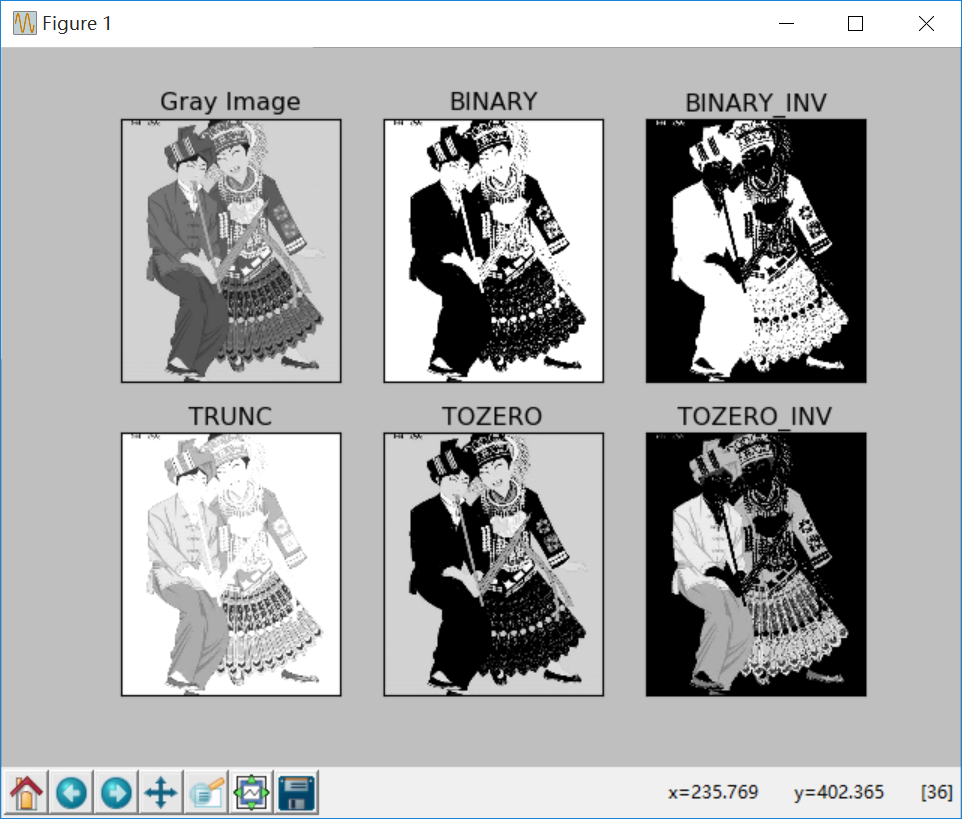（By：Eastmount 2018-10-30 晚上10点 https://blog.csdn.net/Eastmount/）

©️2019 CSDN 皮肤主题: 书香水墨 设计师: CSDN官方博客